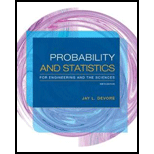# a. Let X 1 have a chi-squared distribution with parameter v 1 (see Section 4.4), and let X 2 be independent of X 1 and have a chi-squared distribution with parameter v 2 . Use the technique of Example 5.22 to show that X 1 + X 2 has a chi-squared distribution with parameter v 1 + v 2 . b. In Exercise 71 of Chapter 4, you were asked to show that if Z is a standard normal rv, then Z 2 has a chi-squared distribution with v = 1. Let Z 1 , Z 2 , . . . , Zn be n independent standard normal rv’s. What is the distribution of Z 1 2 +…+ Zn 2 ? Justify your answer. c. Let X 1 , … , X n be a random sample from a normal distribution with mean µ and variance σ 2 . What is the distribution of the sum Y = ∑ i = 1 n [ ( X i − μ ) / σ ] 2 ? Justify your answer.### Probability and Statistics for Eng...

9th Edition
Jay L. Devore
Publisher: Cengage Learning
ISBN: 9781305251809### Probability and Statistics for Eng...

9th Edition
Jay L. Devore
Publisher: Cengage Learning
ISBN: 9781305251809

#### Solutions

Chapter
Section
Chapter 5, Problem 91SE
Textbook Problem

## Expert Solution

### Want to see the full answer?

Check out a sample textbook solution.See solution

### Want to see this answer and more?

Experts are waiting 24/7 to provide step-by-step solutions in as fast as 30 minutes!*

See Solution

*Response times vary by subject and question complexity. Median response time is 34 minutes and may be longer for new subjects.